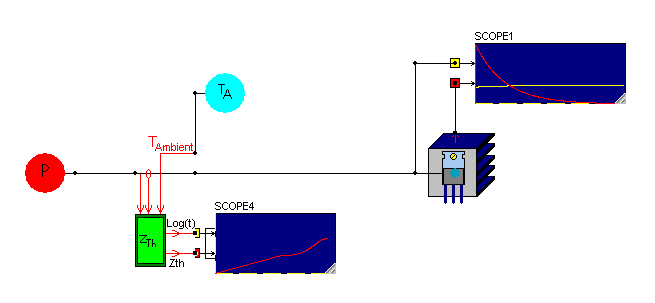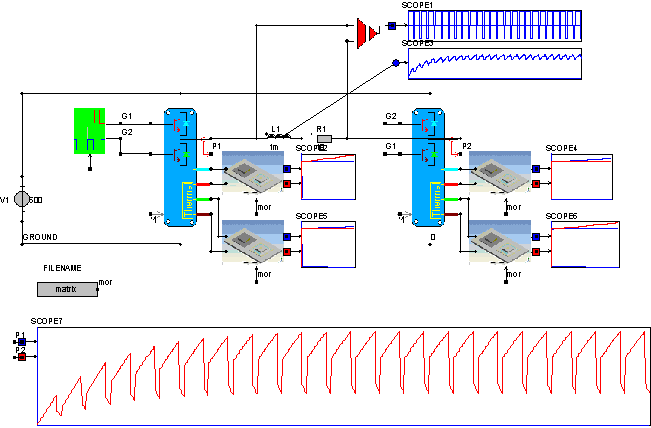• Introduction
• What is in this manual
• What is Caspoc
• User interface
• Introduction
• Starting
• Simulation
• Editing
• Viewing and printing
• Getting Started
• Basic editing
• Simulation in the time domain
• Basic User Interface Topics
• Editing
• Simulation
• Viewing
• Library
• Reports
• Project management
• Circuit and Block Diagram Components
• Introduction
• Cscript and user defined functions
• Component parameters
• Modeling Topics
• Introduction
• Power Electronics
• Semiconductors
• Electrical Machines
• Electrical drives
• Power Systems
• Mechanical Systems
• Thermal Systems
• Magnetic Circuits
• Green Energy
• Coupling to FEM
• Experimenter
• Analog hardware description language
• Embedded C code Export
• Coupling to Spice
• Small Signal Analysis
• Matlab coupling
• Tips and tricks
• Appendices

## Thermal modeling overview.

Thermal modeling is required to simulate the thermal behavior in a system as well as to predict the temperature dependent losses in semiconductors. There are two methods for modeling thermal systems. A library with ready to use thermal models is available. Second there is a coupling to a reduced order model which is supplied from Ansys. Depending on the accuracy of the thermal model the ready to use models can be applied, or the reduced order model can be used. The library components can be found in the section Components/Library/ThermalThe thermal model is set up as a circuit model. The thermal models have thermal nodes on the left and right side. You can combine various thermal models by simply connecting them.The scope in the figure above shows the temperature rise on TO220 package between the TO220 package and the isolation layer.

Basic thermal models
The figure below shows an assembled heat sink and the thermal impedance of this heat sink. The power is injected by a constant power source P. The thermal impedance is measured by the block Zth. On the right side ofthis block you can connect a scope where the upper output is the time in logarithmic scale and the second output is the thermal impedance.From the thermal nodes the temperature can be measured. As can be seen in the scope above, the temperature on the heat sink is shown in scope 1 by the yellow line. The loss disipated in the heat sink is indicated by the red line in scope 1. This dissipated power is exported as a numerical signal at the top of the assembled heat sink model. Be carefull that you can only use this block diagram node for reading the numerical value of the dissipated losses. It can not be connected to other thermal models.

Reduced Order Models
The MOR model is a Reduced Order Model that is directly calculated form the Finite Element Matrix in Ansys using the MOR method. Therefore it incorporates the complete thermal model set up in Ansys and no external models are required. Only one parameter, the ambient temperature and the corresponding Reduced Order Model name have to be defined.